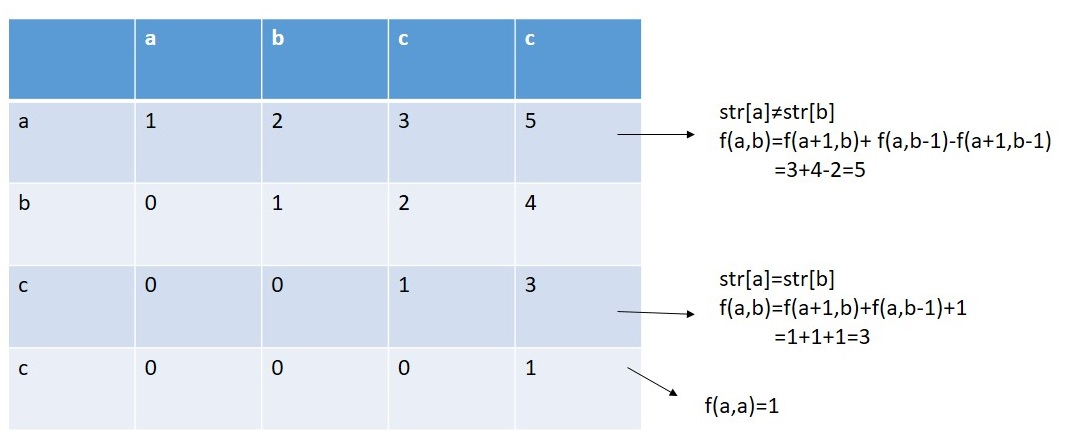# Count the number of palindromic subsequences in a given string

Here, we are going to learn how to count the number of palindromic subsequences in a given string using Dynamic programming?
Submitted by Souvik Saha, on March 31, 2020

Problem statement:

Given a string you have to count the total number of palindromic subsequences in the giving string and print the value.

```    Input:
T Test case
T no of input string will be given to you.

E.g.
3

abc
aa
abcc

Constrain
1≤ length (string) ≤100

Output:
Print the count of the palindromic sub sequences from the given string.
```

Example

```    T=3

Input:
abc

Output:
3 ("a", "b", "c")

Input:
aa

Output:
3 ("a", "a", "aa")

Input:
abcc

Output:
5 ("a", "b", "c", "c", "cc")
```

Explanation with example:

Let there is a string str.

Now possible arrangements are:

1. Single middle characters like aba
2. Paired middle characters like bb

Let, f(a,b) = count of number of palindromic subsequences from index a to index b.

Considering the above two facts:

1. If there is only one character then f(a,a) = 1
2. If there is a substring starting from index a to index b then,
1. If str[a] and str[b] both are same then
f(a,b)=f(a+1,b)+f(a,b-1)+1
2. If str[a] and str[b] both are not same then
f(a,b)=f(a+1,b)+f(a,b-1)-f(a+1,b-1)

For, str = abbaaFrom the above, it is understandable that one function is called repeatedly so for the large input the repetition will be very high. Because of this problem we are using a Dynamic programming approach to avoid repetition. We are using the memorization process to solve the problem.

Problem solution:

Recursive algorithm:

```int Function(str,pos,l):
if str.length()== l
return
for a=pos to str.length()-l
if str[a]==str[a+l-1]
return Function(str,a,l-1)+Function(str,a+1,l-1)+1
else
return Function(str,a,l-1)+Function(str,a+1,l-1)-Function(str,a+1,l-2)
```

DP conversion:

```int Function(str,n):
for len=1 to str.length()
for a=0 to str.length()-len
b=pos+len-1
if str[a]==str[b]
arr[a][b]=arr[a+1][b]+ arr[a][b-1]+1
else
arr[a][b]=arr[a+1][b]+arr[a][b-1]-arr[a+1][b-1]
return arr[len-1]
```

C++ Implementation:

```#include <bits/stdc++.h>
using namespace std;

int count_palindrome(string str)
{
int len = str.length();
int arr[len][len] = { 0 };
for (int i = 0; i < len; i++) {
arr[i][i] = 1;
}
for (int l = 2; l <= len; l++) {
for (int i = 0; i <= len - l; i++) {
int j = i + l - 1;
if (str[i] == str[j]) {
arr[i][j] = arr[i + 1][j] + arr[i][j - 1] + 1;
}
else {
arr[i][j] = arr[i + 1][j] + arr[i][j - 1] - arr[i + 1][j - 1];
}
}
}
return arr[len - 1];
}

int main()
{
int t;
cout << "Test Case : ";
cin >> t;
while (t--) {
cout << "Enter the string : ";
string str;
cin >> str;
cout << "Number of palindromic subsequences are : " << count_palindrome(str) << endl;
}

return 0;
}
```

Output

```Test Case : 3
Enter the string : abc
Number of palindromic subsequences are : 3
Enter the string : aaa
Number of palindromic subsequences are : 7
Enter the string : abcc
Number of palindromic subsequences are : 5
```